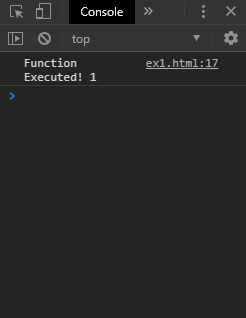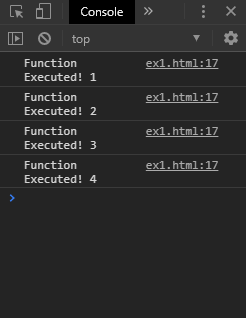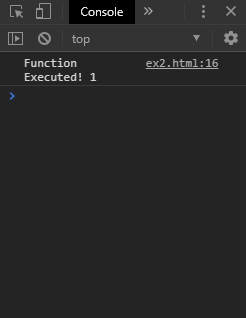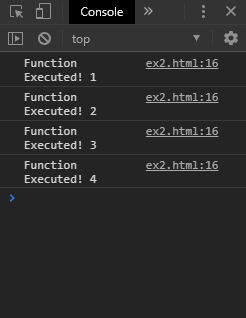# How to execute setInterval function without delay for the first time in JavaScript ?

• Last Updated : 19 Nov, 2019

The setInterval() method always invokes the function after the delay for the first time using two approaches:

Method 1: Calling the function once before executing setInterval: The function can simply be invoked once before using the setInterval function. This will execute the function once immediately and then the setInterval() function can be set with the required callback.
A new function is created which encloses the invoking of the function first and then calls the setInterval() function next. This will help to simulate the setInterval() function without a delay for the first time.

Example:

 ```<``html``>`` ` `<``head``>``    ``<``title``>``        ``How to execute setInterval function without``        ``delay for the first time in JavaScript ?``    `````` ` `<``body``>``    ``<``h1` `style``=``"color: green"``>``        ``GeeksforGeeks``    ````     ` `    ``<``b``>``        ``Execute the setInterval function``        ``without delay the first time``    ````     ` `    ``<``p``>``        ``Click on the button to execute the``        ``setInterval() function without delay.``    ````     ` `    ``<``button` `onclick``=``"startSetInterval()"``>``        ``Start immediate setInterval``    ````     ` `    ``<``script` `type``=``"text/javascript"``>``         ` `        ``let count = 1;``     ` `        ``function exampleFunction() {``            ``console.log('Function Executed! ' + count);``            ``count = count + 1;``        ``}``     ` `        ``function noDelaySetInterval(func, interval) {``            ``func();``            ``return setInterval(func, interval);``        ``}``     ` `        ``function startSetInterval() {``            ``noDelaySetInterval(exampleFunction, 3000);``        ``}``    `````` ` ``

Output:

• Immediately after clicking the button:• After waiting for 3 seconds:Method 2: Using an immediately invoking function inside setInterval function: An Immediately-invoked Function Expression (IIFE) is a type of function that immediately gets invoked after its declaration. This property can be used in the callback of the setInterval() function, as it would get immediately executed once and then the actual setInterval() with this function will start after the specified delay. This will help to simulate the setInterval() function without a delay for the first time.

Example:

 ```<``html``>`` ` `<``head``>``    ``<``title``>``        ``Execute the setInterval function``        ``without delay the first time``    `````` ` `<``body``>``    ``<``h1` `style``=``"color: green"``>``        ``GeeksforGeeks``    ````     ` `    ``<``b``>``        ``Execute the setInterval function``        ``without delay the first time``    ````     ` `    ``<``p``>``        ``Click on the button to execute the``        ``setInterval() function without delay.``    ````     ` `    ``<``button` `onclick``=``"startSetInterval()"``>``        ``Start immediate setInterval``    ````     ` `    ``<``script` `type``=``"text/javascript"``>``     ` `        ``function startSetInterval() {``             ` `            ``let count = 1;``             ` `            ``setInterval(function exampleFunction() {``                ``console.log('Function Executed! ' + count);``                ``count = count + 1;``         ` `                ``return exampleFunction;``            ``}(), 3000);``        ``}``    `````` ` ``

Output:

• Immediately after clicking the button:• After waiting for 3 seconds:My Personal Notes arrow_drop_up